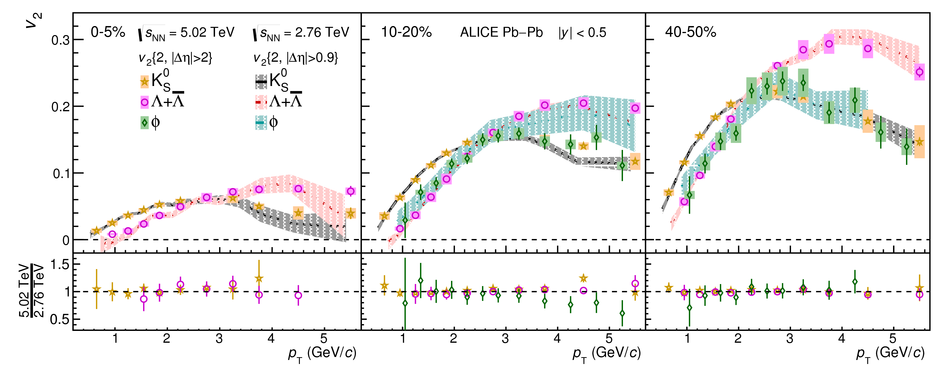# Anisotropic flow of identified particles in Pb-Pb collisions at $\mathbf{\sqrt{{\textit s}_{\rm NN}}}=5.02$ TeV

The elliptic ($v_2$), triangular ($v_3$), and quadrangular ($v_4$) flow coefficients of $\pi^{\pm}$, ${\rm K}^{\pm}$, ${\rm p+\overline{p}}$, ${\Lambda+\overline{\Lambda}}$, ${\rm K}^{\rm 0}_{\rm S}$, and the $\phi$-meson are measured in Pb-Pb collisions at $\sqrt{s_{\rm NN}}=5.02$ TeV. Results obtained with the scalar product method are reported for the rapidity range $\vert y \vert <~$ 0.5 as a function of transverse momentum, $p_\text{T}$, at different collision centrality intervals between 0-70%, including ultra-central (0-1%) collisions for $\pi^{\pm}$, ${\rm K}^{\pm}$, and ${\rm p+\overline{p}}$. For $p_\text{T} <~ 3$ GeV$\kern-0.05em/\kern-0.02em c$, the flow coefficients exhibit a particle mass dependence. At intermediate transverse momenta ($3<~p_\text{T}<~$~8-10 GeV$\kern-0.05em/\kern-0.02em c$), particles show an approximate grouping according to their type (i.e., mesons and baryons). The $\phi$-meson $v_2$, which tests both particle mass dependence and type scaling, follows ${\rm p+\overline{p}}$ $v_2$ at low $p_\text{T}$ and $\pi^{\pm}$ $v_2$ at intermediate $p_\text{T}$. The evolution of the shape of $v_{\rm n}(p_{\mathrm{T}})$ as a function of centrality and harmonic number $n$ is studied for the various particle species. Flow coefficients of $\pi^{\pm}$, ${\rm K}^{\pm}$, and ${\rm p+\overline{p}}$ for $p_\text{T}<~3$ GeV$\kern-0.05em/\kern-0.02em c$ are compared to iEBE-VISHNU and MUSIC hydrodynamical calculations coupled to a hadronic cascade model (UrQMD). The iEBE-VISHNU calculations describe the results fairly well for $p_\text{T} <~ 2.5$ GeV$\kern-0.05em/\kern-0.02em c$, while MUSIC calculations reproduce the measurements for $p_\text{T} <~ 1$ GeV$\kern-0.05em/\kern-0.02em c$. A comparison to $v_{\rm n}$ coefficients measured in Pb-Pb collisions at $\sqrt{s_{\rm NN}}$ = 2.76 TeV is also provided.

Figures

## Figure 1

 Illustration of reconstruction and $v_2$ measurement for the $\phi$-meson. The reconstruction of the $\phi$-meson and extraction of $N^{\rm sig}$ and $N^{\rm bg}$ are shown in the upper panel. A fit of Eq.~\ref{eq:invmassfit} to data is presented in the lower panel.## Figure 2

 Centrality dependence of \vtwopt{} for \pipm{}, \kapm{}, p+\pbar{}, \lambdas{}, \kanull{}, and the $\phi$-meson. Statistical and systematic uncertainties are shown as bars and boxes, respectively.## Figure 3

 Centrality dependence of \vthreept{} for \pipm{}, \kapm{}, p+\pbar{}, \lambdas{}, and \kanull{}. Statistical and systematic uncertainties are shown as bars and boxes, respectively.## Figure 4

 Centrality dependence of \vfourpt{} for \pipm{}, \kapm{}, and p+\pbar{}. Statistical and systematic uncertainties are shown as bars and boxes, respectively.## Figure 5

 The \pt-differential $v_2$ of \pipm{}, \kapm{}, p+\pbar{}, \lambdas{}, \kanull{}, and the $\phi$-meson for various centrality classes. Statistical and systematic uncertainties are shown as bars and boxes, respectively.## Figure 6

 The \pt-differential $v_3$ of \pipm{}, \kapm{}, p+\pbar{}, \lambdas{}, and \kanull{} for various centrality classes. Statistical and systematic uncertainties are shown as bars and boxes, respectively.## Figure 7

 The \pt-differential $v_4$ of \pipm{}, \kapm{}, and p+\pbar{} for various centrality classes. Statistical and systematic uncertainties are shown as bars and boxes, respectively.## Figure 8

 The $\pt/n_{\rm q}$ dependence of $v_2/n_{\rm q}$ of \pipm{}, \kapm{}, p+\pbar{}, \lambdas{}, \kanull{}, and the $\phi$-meson for various centrality classes. Statistical and systematic uncertainties are shown as bars and boxes, respectively.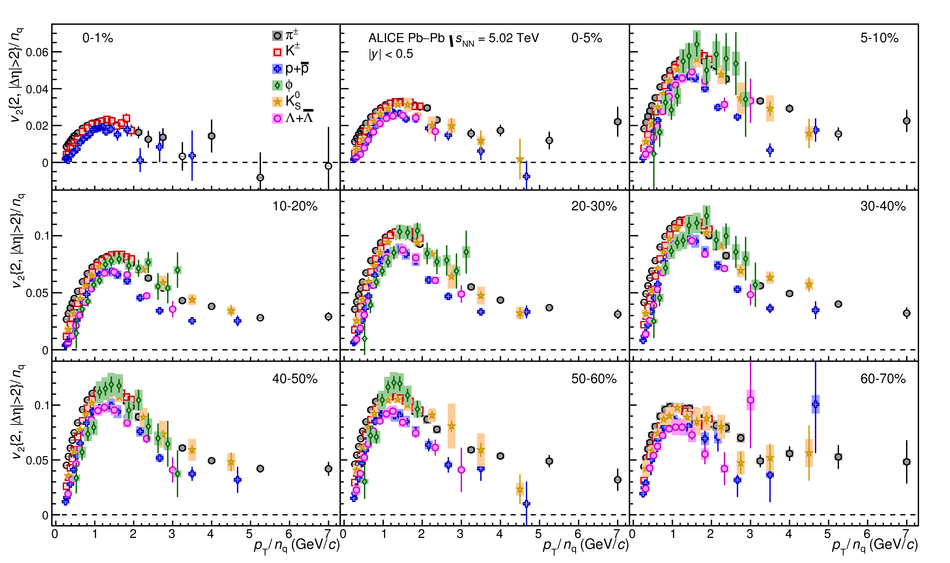## Figure 9

 The $\pt/n_{\rm q}$ dependence of $v_3/n_{\rm q}$ of \pipm{}, \kapm{}, p+\pbar{}, \lambdas{}, and \kanull{} for various centrality classes. Statistical and systematic uncertainties are shown as bars and boxes, respectively.## Figure 10

 The $\pt/n_{\rm q}$ dependence of $v_4/n_{\rm q}$ of \pipm{}, \kapm{}, and p+\pbar{} for various centrality classes. Statistical and systematic uncertainties are shown as bars and boxes, respectively.## Figure 11

 The $\pt$-differential $v_2$ (top), $v_3$ (middle), and $v_4$ (bottom) of \pipm{} for the 0--5\%, 10--20\%, and 40--50\% centrality classes compared to hydrodynamical calculations from MUSIC model using IP-Glasma initial conditions (magenta)~ and the iEBE-VISHNU hybrid model using AMPT (orange) or \protect\trento{}~(cyan) initial conditions~. Statistical and systematic uncertainties of the data points are shown as bars and boxes, respectively. The uncertainties of the hydrodynamical calculations are depicted by the thickness of the curves. The ratios of the measured $v_{\rm n}$ to a fit to the hydrodynamical calculations are also presented for clarity.## Figure 12

 The $\pt$-differential $v_2$ (top), $v_3$ (middle), and $v_4$ (bottom) of \kapm{} for the 0--5\%, 10--20\%, and 40--50\% centrality classes compared to hydrodynamical calculations from MUSIC model using IP-Glasma initial conditions (magenta)~ and the iEBE-VISHNU hybrid model using AMPT (orange) or \protect\trento{}~(cyan) initial conditions~. Statistical and systematic uncertainties of the data points are shown as bars and boxes, respectively. The uncertainties of the hydrodynamical calculations are depicted by the thickness of the curves. The ratios of the measured $v_{\rm n}$ to a fit to the hydrodynamical calculations are also presented for clarity.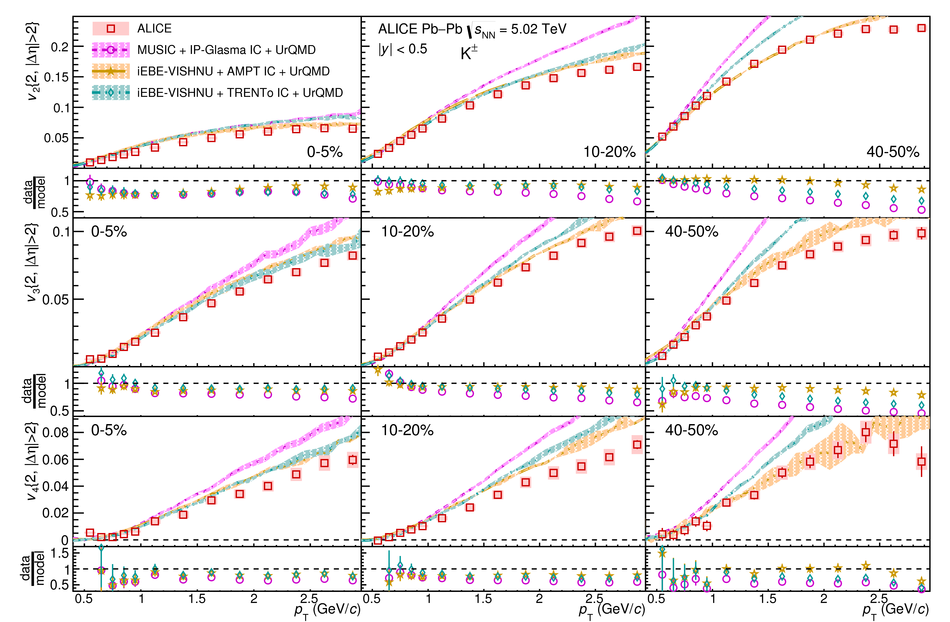## Figure 13

 The $\pt$-differential $v_2$ (top), $v_3$ (middle), and $v_4$ (bottom) of p+\pbar{} for the 0--5\%, 10--20\%, and 40--50\% centrality classes compared to hydrodynamical calculations from MUSIC model using IP-Glasma initial conditions (magenta)~ and the iEBE-VISHNU hybrid model using AMPT (orange) or \protect\trento{}~(cyan) initial conditions~. Statistical and systematic uncertainties of the data points are shown as bars and boxes, respectively. The uncertainties of the hydrodynamical calculations are depicted by the thickness of the curves. The ratios of the measured $v_{\rm n}$ to a fit to the hydrodynamical calculations are also presented for clarity.## Figure 14

 Centrality dependence of $\vtwopt{}_{\rm ratio~to~20-30\%}$ for \pipm{}, \kapm{}, p+\pbar{}, and inclusive charged particles . Statistical and systematic uncertainties are shown as bars and boxes, respectively.## Figure 15

 Centrality dependence of $\vthreept{}_{\rm ratio~to~20-30\%}$ for \pipm{}, \kapm{}, p+\pbar{}, and inclusive charged particles . Statistical and systematic uncertainties are shown as bars and boxes, respectively.## Figure 16

 Centrality dependence of $\vtwopt{}_{\rm ratio~to~20-30\%}$ for \pipm{} (upper panels), \kapm{} (middle panels), and p+\pbar{} (lower panels) compared to hydrodynamical calculations from the MUSIC model using IP-Glasma initial conditions (magenta)~, the iEBE-VISHNU hybrid model using AMPT (orange) or \protect\trento{}~(cyan) initial conditions~. Statistical and systematic uncertainties of the data points are shown as bars and boxes, respectively.## Figure 17

 Centrality dependence of \vtwomax{} (left) and \vthreemax{} (right) divided by number of constituent quarks, $n_{\rm q}$, for \pipm{}, p+\pbar{}, \lambdas{}, and \kanull{}. Points are slightly shifted along the horizontal axis for better visibility in both panels. Statistical and systematic uncertainties are shown as bars and boxes, respectively.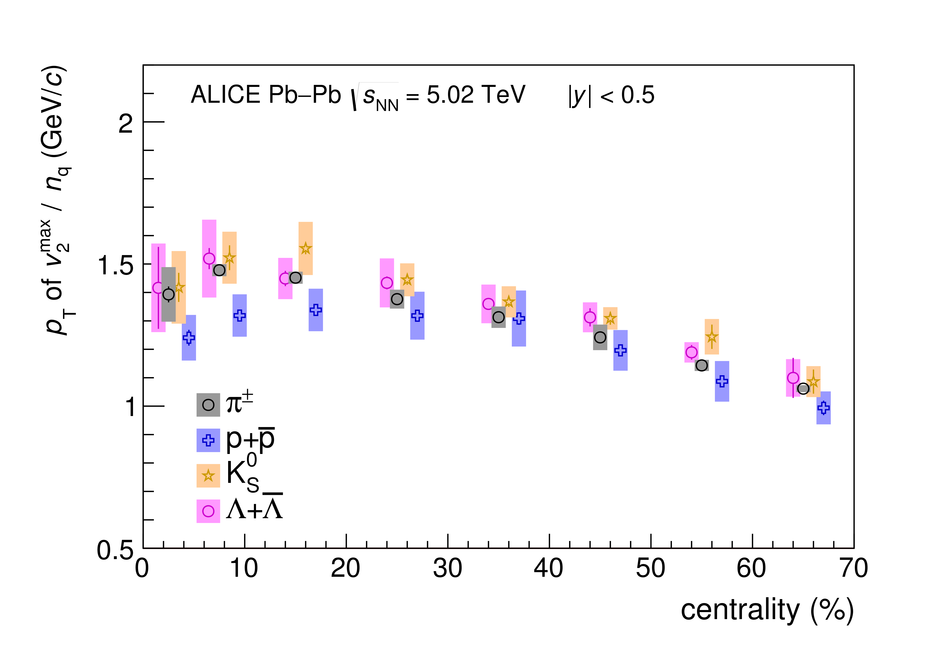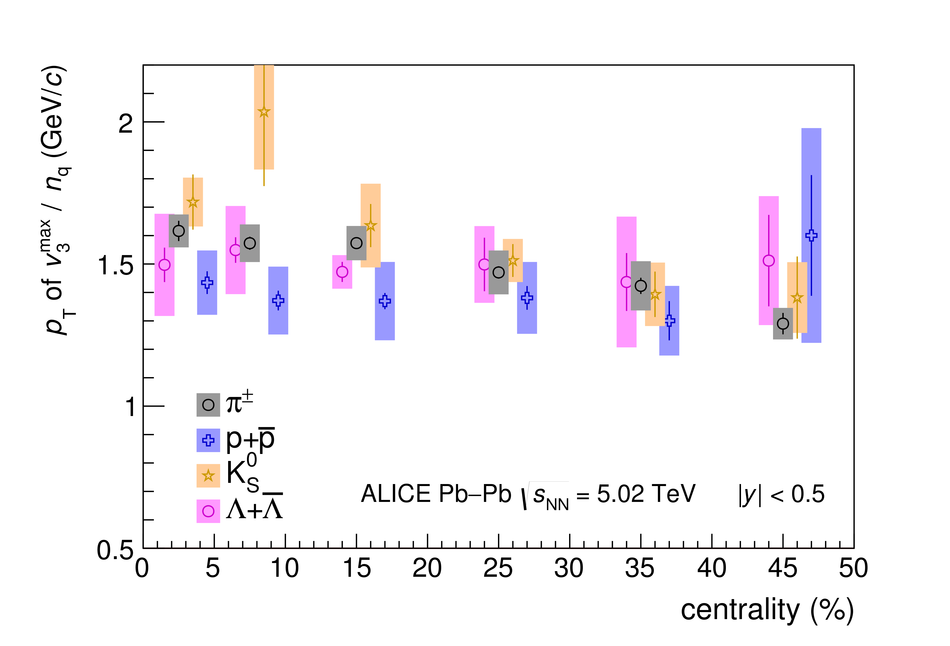## Figure 18

 Centrality dependence of $|v_{\rm n}|^{1/{\rm n}} / \pt{}$ of inclusive charged particles , \pipm{}, \kapm{}, p+\pbar{}, \lambdas{}, \kanull{}, and the $\phi$-meson for $n=2$ (upper panels) and $n=3$ (lower panels). Statistical and systematic uncertainties are shown as bars and boxes, respectively.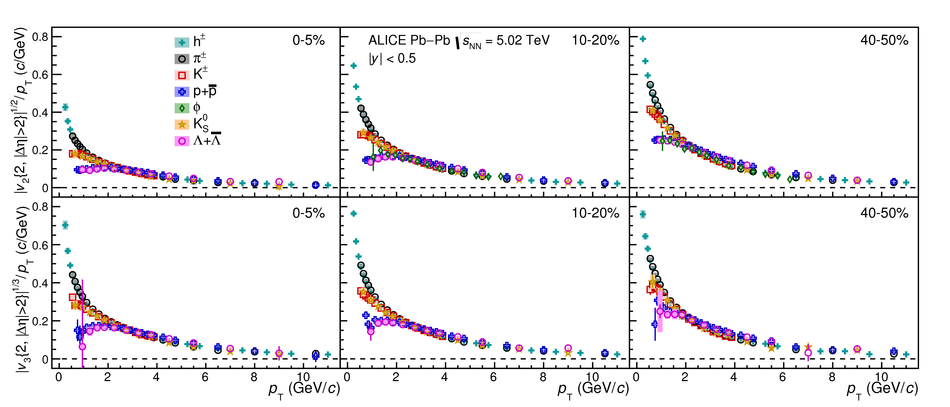## Figure 19

 Centrality dependence of $v_3/\vert v_2\vert^{3/2}$ for inclusive charged particles , \pipm{}, \kapm{}, and p+\pbar{}. Statistical and systematic uncertainties are shown as bars and boxes, respectively.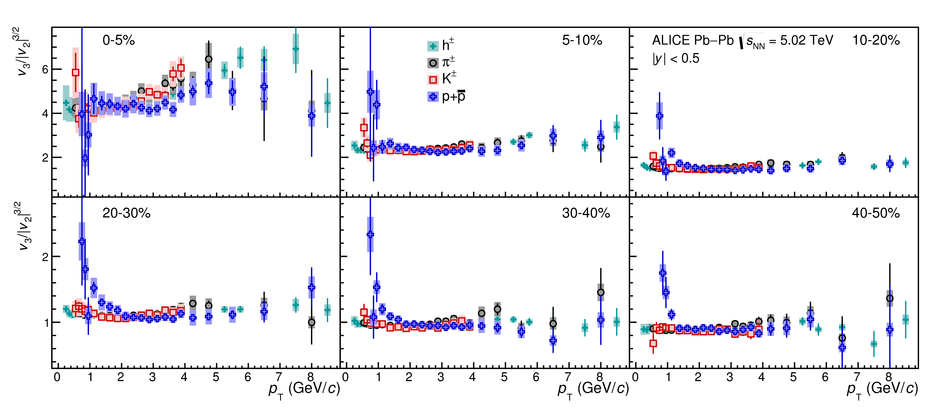## Figure 20

 Centrality dependence of $v_4/\vert v_2\vert^{4/2}$ for inclusive charged particles , \pipm{}, \kapm{}, and p+\pbar{}. Statistical and systematic uncertainties are shown as bars and boxes, respectively.## Figure 21

 Centrality dependence of $v_4/\vert v_3\vert^{4/3}$ for inclusive charged particles , \pipm{}, \kapm{}, and p+\pbar{}. Statistical and systematic uncertainties are shown as bars and boxes, respectively.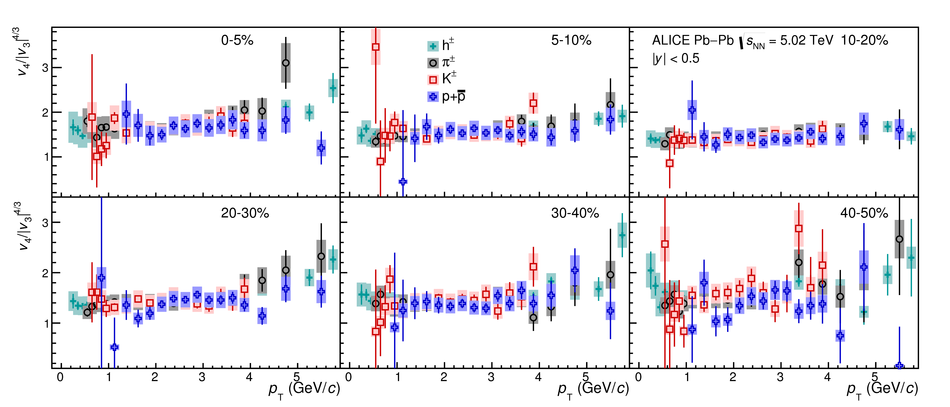## Figure 22

 The $\pt$-differential $v_2$ (top), $v_3$ (middle), and $v_4$ (bottom) of \pipm{}, \kapm{}, and p+\pbar{} compared to ALICE measurements performed in Pb--Pb collisions at \sqrtSnn{} = 2.76~TeV (coloured bands)~ for the 0--5\%, 10--20\%, and 40--50\% centrality classes. For the measurements at \sqrtSnn{} = 5.02~TeV, statistical and systematic uncertainties are shown as bars and boxes, respectively. For the measurements at \sqrtSnn{} = 2.76~TeV, the thickness of the bands corresponds to the quadratic sum of statistical and systematic uncertainties. The ratios of measurements at \sqrtSnn{} = 5.02~TeV to a cubic spline fit to the measurements at \sqrtSnn{} = 2.76~TeV are also presented for clarity.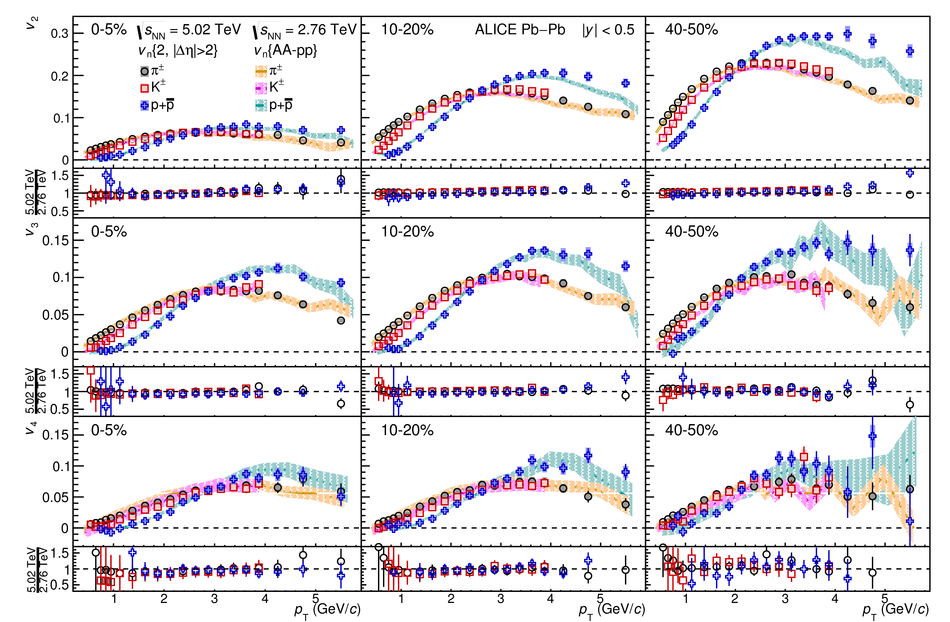## Figure 23

 The $\pt$-differential $v_2$ of \lambdas{}, \kanull{}, and the $\phi$-meson compared to ALICE measurements performed in Pb--Pb collisions at \sqrtSnn{} = 2.76~TeV (coloured bands)~ for the 0--5\%, 10--20\%, and 40--50\% centrality classes. For the measurements at \sqrtSnn{} = 5.02~TeV, statistical and systematic uncertainties are shown as bars and boxes, respectively. For the measurements at \sqrtSnn{} = 2.76~TeV, the thickness of the bands corresponds to the quadratic sum of statistical and systematic uncertainties. The ratios of measurements at \sqrtSnn{} = 5.02~TeV to a cubic spline fit to the measurements at \sqrtSnn{} = 2.76~TeV are also presented for clarity.